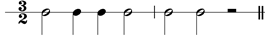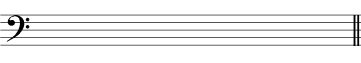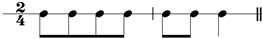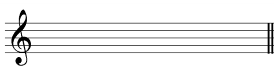In the grade two Trinity exam, you may be asked to compose a short melody to a given rhythm. You will normally be given some guidance on the notes you need to use, for example:

• use notes of the tonic triad
• use the first five degrees of the scale

In this question the examiner will be looking for the following:

• correct notation (stem direction, nicely drawn notes)
• accurate copying of the given rhythm
• correctly written key signature
• correct selection of notes (take notice of the clef!)

Here is an example.

Write a tune to the given rhythm using the notes of the tonic triad. Use a key signature and finish on the tonic.

G major

First of all, work out which notes you are supposed to use. The key is G major, so the notes of the tonic triad are G, B and D. The tonic is G, so that is the note we need to end on. You can use the notes in any register – high or low, but your melody will sound better if you avoid lots of enormous leaps!

Here is a different type of question.

Write a tune to the given rhythm using the first five notes of the scale. Use a key signature and finish on the tonic.

D minor

The first five notes in the scale of D minor are D-E-F-G-A and the tonic is D. Try to use all the notes.

## Composition Exercises

a. Write a tune to the given rhythm using the notes of the tonic triad. Use a key signature and finish on the tonic.

E minorb. Write a tune to the given rhythm using the first five notes of the scale. Use a key signature and finish on the tonic.

F major